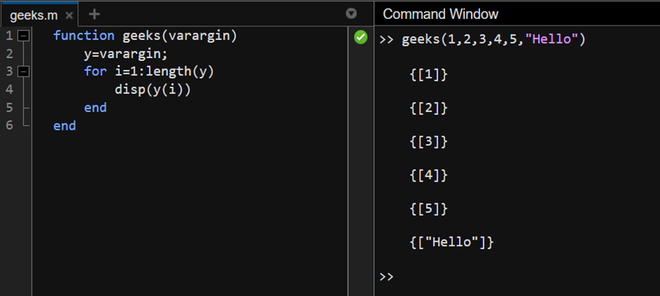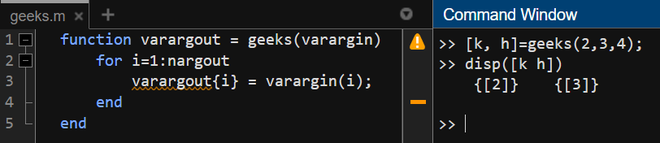Open in App
Not now

# Function With Variable Number of Input Arguments in MATLAB

• Last Updated : 29 Dec, 2022

MATLAB functions have the functionality that many other programming languages lack. A MATLAB function can take a variable number of input arguments, without the use of arrays or any additional functionality. The way to go about this in MATLAB is by using the varargin – which can be interpreted as VARiable ARGument INput. Now, the way to use varargin is in all lowercase and it must be passed as an input argument in the function declaration after all other input parameters.

## Syntax

function fun1 = fun(var1, var2, …, varargin)

—function body—

end

Varargin converts the input arguments to a cell array and thus, making it accessible within the scope of the function only.

See the following examples to understand the same.

## Example 1

In this example, we shall create a function that displays all the input arguments.

## Matlab

 `% function``function` `geeks(varargin)``    ``y=varargin;``    ``for` `i=1:length(y)``        ``disp(y(i))``    ``end``end`

We create a copy of the above varargin in the y variable and then, access it as an ordinary cell array.

Output:## Example 2

In this example, we assign the output variables to the given input variables. The assignment depends on a number of output variables passed.

## Matlab

 `% function``function` `varargout = geeks(varargin)``    ``for` `i=1:nargout``        ``varargout{i} = varargin(i);``    ``end``end`

In this function, we iterate through the length of output variables using the nargout keyword and assign each of the output variables, the respective input variable in sequence.

Output:My Personal Notes arrow_drop_up